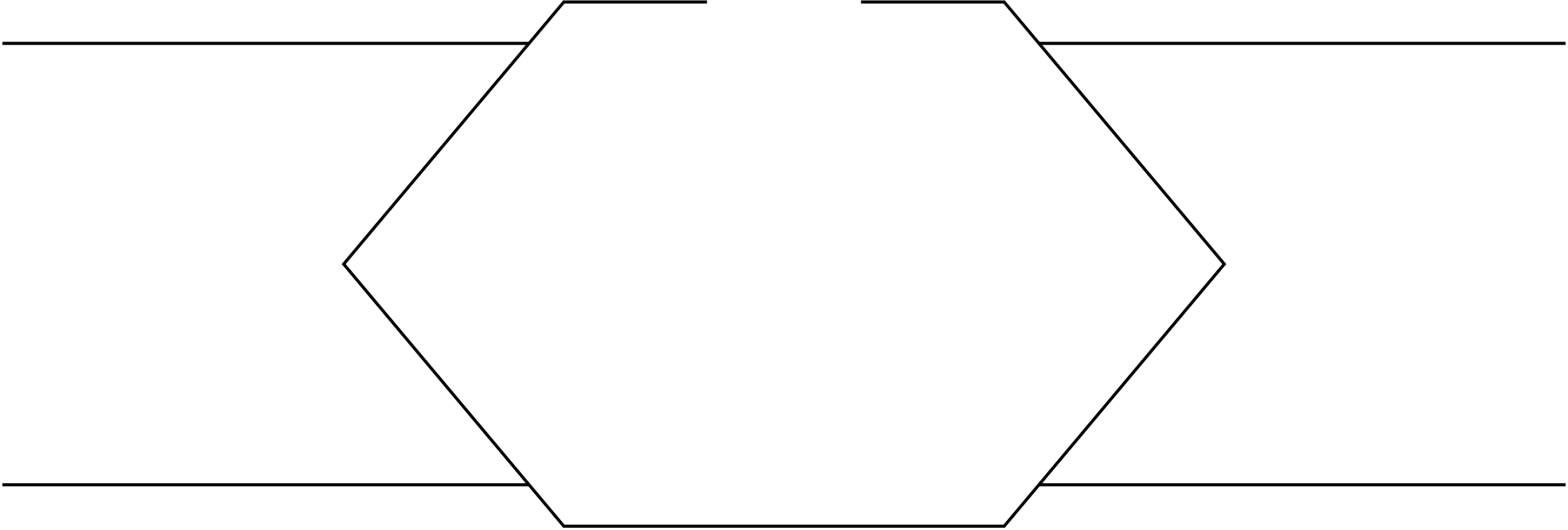Rating : ⭐⭐⭐⭐⭐
Price : \$10.99
Language:EN
Pages: 2

# The algorithm based parametric represen-tation the ellipseGRAPHIC GEMS III Edited by DAVID KIRK

The fourth, sixth, and seventh Gems present clever improvements upon previous Gems. The fourth and sixth Gems discuss efficient computation of the intersection between two lines, while the seventh Gem discusses the construction of circles tangent to other figures, and related problems.

GRAPHICS GEMS III Edited by DAVID KIRK 163

Sometimes an arc of a circle or of an ellipse is a better choice than a cubic spline for representing a particular curved shape. Because circles and ellipses are inherently simpler curves than cubics, the algorithms for generating them should also be simpler. This is chiefly why conic splines are popular in applications such as the generation of font outlines, where drawing speed is of critical importance.

This note describes an algorithm for generating points along an ellipti-cal arc. The points are separated by a fixed angular increment specified in radians of elliptical arc. The algorithm is based on a parametric represen-tation of the ellipse. It is particularly inexpensive in terms of the amount of computation required. Only a few integer (or fixed-point) shifts, addi-tions, and subtractions are needed to generate each point—without compromising accuracy.

How It Works